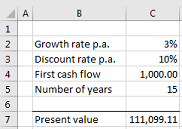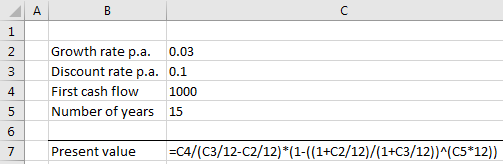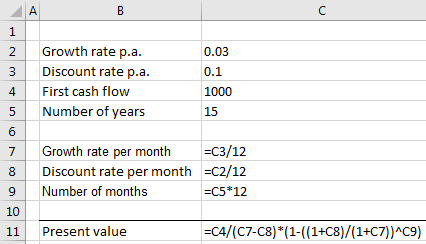Financial maths competition
Try our live financial maths quiz / competition

#XL-Excellence® - Examples - Formula complexity

Overly complex formulae can be an area of spreadsheet weakness. The Add-In can detect such formulae.

Consider the following example.

The spreadsheet below calculates the present value of a series of monthly cash flows. The calculation inputs are in cells C2,C3,C4 & C5. The calculation’s result is shown in cell C7.The calculation above spans 15 years of monthly cash flows. The first cash flow to be received is 1,000 and then grows at the rate of 3 percent per year. To present-value the cash flows a discount rate of 10 percent per year is applied.

Following is an XL-Excellence® report on the calculation showing a heatmap coloured on the basis of formula complexity.Note that the formula in cell C7 is coloured orange. Complexity is defined in terms of formula length. Formulas less than a “short” threshold are shown in green. “Medium” formulas are orange and “Long” formulas are shown in red. The length of “short” , “medium” and “long” formulas can be defined by selecting the Settings | Formulae choices in the ribbon. The thresholds used in the example shown here are as follows ..Opening the original workbook and pressing the CTRL + ` keys reveals the underlying “orange” formula. The formula is, indeed, fairly complex.It is best to break complex formulas into simpler parts. Having done that and re-generating the heatmap we see ..Instead of one complex (orange) formulas we now have more – but simpler – green formulas.
How was that achieved?We have broken the calculation into simpler components. In particular we calculate monthly
data from yearly and then pass the result into a simpler final formula.

Pressing CTRL + ` shows that the resulting formulas are indeed simpler than the original.If you wish to experiment with the examples discussed above you can download the examples by choosing from the Links section.